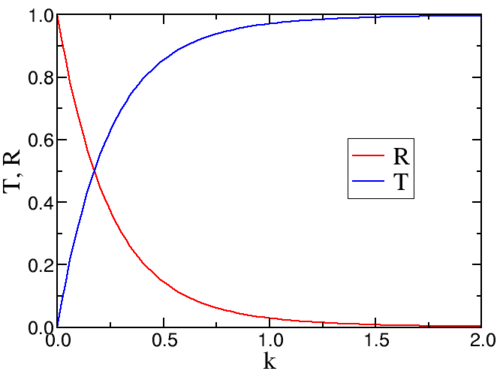# 6.2: Potential step

$$\newcommand{\vecs}{\overset { \rightharpoonup} {\mathbf{#1}} }$$ $$\newcommand{\vecd}{\overset{-\!-\!\rightharpoonup}{\vphantom{a}\smash {#1}}}$$$$\newcommand{\id}{\mathrm{id}}$$ $$\newcommand{\Span}{\mathrm{span}}$$ $$\newcommand{\kernel}{\mathrm{null}\,}$$ $$\newcommand{\range}{\mathrm{range}\,}$$ $$\newcommand{\RealPart}{\mathrm{Re}}$$ $$\newcommand{\ImaginaryPart}{\mathrm{Im}}$$ $$\newcommand{\Argument}{\mathrm{Arg}}$$ $$\newcommand{\norm}{\| #1 \|}$$ $$\newcommand{\inner}{\langle #1, #2 \rangle}$$ $$\newcommand{\Span}{\mathrm{span}}$$ $$\newcommand{\id}{\mathrm{id}}$$ $$\newcommand{\Span}{\mathrm{span}}$$ $$\newcommand{\kernel}{\mathrm{null}\,}$$ $$\newcommand{\range}{\mathrm{range}\,}$$ $$\newcommand{\RealPart}{\mathrm{Re}}$$ $$\newcommand{\ImaginaryPart}{\mathrm{Im}}$$ $$\newcommand{\Argument}{\mathrm{Arg}}$$ $$\newcommand{\norm}{\| #1 \|}$$ $$\newcommand{\inner}{\langle #1, #2 \rangle}$$ $$\newcommand{\Span}{\mathrm{span}}$$$$\newcommand{\AA}{\unicode[.8,0]{x212B}}$$

Consider a potential step

V(x) = \left\{\begin{aligned} & V_0 && x < a \\ & V_1 && x > a \end{aligned} \right. \label{6.3}Figure $$\PageIndex{1}$$: The step potential discussed in the text

Let me define

\begin{align} k_0 &= \sqrt{ \dfrac{2 m}{ ℏ^ 2} ( E − V_0 )} , \label{6.4} \\[5pt] k_1 &= \sqrt{ \dfrac{2 m}{ℏ^ 2} ( E − V_1 )} . \label{6.5} \end{align}

I assume a beam of particles comes in from the left,

$ϕ(x) = A_0 e^{ i k_0 x} \, \, \text{for } x < 0 . \label{6.6}$

At the potential step the particles either get reﬂected back to region I, or are transmitted to region II. There can thus only be a wave moving to the right in region II, but in region I we have both the incoming and a reﬂected wave,

\begin{align} ϕ_I (x) &= A 0 e^{ i k_0 x} + B_0 e^{ − i k_0 x} , \label{6.7} \\[5pt] ϕ_{II} (x) &= A_1 e^{ i k_1 x} . \label{6.8} \end{align}

We define a transmission ($$T$$) and reﬂection ($$R$$) coefficient as the ratio of currents between reﬂected or transmitted wave and the incoming wave, where we have canceled a common factor

\begin{align} R &= \dfrac{| B_0 |^2}{ | A_0 |^2} \\[5pt] T &= \dfrac{k_1 | A_1 |^2 }{ k_0 | A_0 |^2} . \label{6.9} \end{align}

Even though we have given up normalisability, we still have the two continuity conditions. At $$x= 0$$ these imply, using continuity of $$ϕ$$ and $$\dfrac{d}{dx}ϕ$$,

\begin{align} A_0 + B_0 &= A_1 , \label{6.10} \\[5pt] i k_0 ( A_0 − B_0 ) &= i k_1 A_1 . \label{6.11} \end{align}

We thus find

\begin{align} A_1 &= \dfrac{2 k_0}{ k_0 + k_1 A_0} \label{6.12} \\[5pt] B_0 &= \dfrac{k_0 − k_1 }{k_0 + k_1 A_0} , \label{6.13} \end{align}

and the reﬂection and transmission coefficients can thus be expressed as

\begin{align} R &= \left( \dfrac{k_0 − k_1}{k_0 + k_1} \right)^2 , \label{6.14} \\[5pt] T &= \dfrac{ 4 k_1 k_0}{ ( k_0 + k_1 )^2} . \label{6.15} \end{align}

Notice that $$R+ T= 1$$!Figure $$\PageIndex{2}$$: The transmission and reﬂection coefficients for a square barrier.

In Figure $$\PageIndex{2}$$ we have plotted the behaviour of the transmission and reﬂection of a beam of Hydrogen atoms impinging on a barrier of height 2 meV.

This page titled 6.2: Potential step is shared under a CC BY-NC-SA 2.0 license and was authored, remixed, and/or curated by Niels Walet via source content that was edited to the style and standards of the LibreTexts platform; a detailed edit history is available upon request.